Want to share your content on R-bloggers? click here if you have a blog, or here if you don't.As we have already seen in former posts simple methods can be surprisingly successful in yielding good results (see e.g Learning Data Science: Predicting Income Brackets or Teach R to read handwritten Digits with just 4 Lines of Code).

If you want to learn how some simple mathematics, known as Naive Bayes, can help you find out the sentiment of texts (in this case movie reviews) read on!

The area we are dealing with here is called Natural Language Processing (NLP) and we already had some posts covering it (see Clustering the Bible and Extracting basic Plots from Novels: Dracula is a Man in a Hole).

We do this by using a simplified version of Bayes’ theorem (see Base Rate Fallacy – or why No One is justified to believe that Jesus rose). We will do it from scratch so that you really understand what is going on under the hood… but there are of course also excellent packages which will do it for you, most notably e1071 (on CRAN).

The big idea is to take texts that are already classified as “positive” and “negative” and use Bayes’ theorem to find the probability of words of unknown texts being positive/negative and sum over the probabilities for both categories to see which one “wins”.

Put differently, we find how probable positive/negative words are (basically by counting how frequently they appear in the given texts) and use that knowledge to weigh how positive or negative a new text is (by summing over all of the probabilities of both categories). Words that don’t signal a sentiment appear less often, relatively speaking (if the given texts are not systematically biased) or cancel each other out because they appear in both categories.

For the mathematical details, a quick repetitions of Bayes’ theorem (the vertical dash means “under the condition that”, P stands for probability):

•: appearance of the specific word () given the text is positive/negative () – this is what we know from our given texts.
•: the text is positive/negative () given the word appears () – this (whether the text is positive/negative) is what we want to know.

To calculate one conditional probability from the other we use Bayes’ theorem: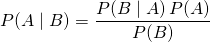Now, for some simplifications to make the calculations easier (and even possible!):

• The first simplification we make is that we eliminate the denominator with its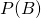because it is the same for both probabilities: the appearance of the respective word is just the same for both expressions.
• The second simplification also eliminates the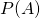in the nominator because it will (at least in our example here) be more or less equal for both probabilities. Why? Because we will use the same amount of positive and negative example reviews (and by proxy about the same amount of positive and negative words).
• Now, we are coming to the “naive” part: we are not only talking about one word but about many words in the given texts. Obviously, their occurrence is not independent of each other, the probability that “good” is in the same text as “great” is higher than “good” being in the same text as “bad”. The problem is that our calculations would become super complicated (in the sense of impossible!) if we tried to model all of those dependencies: so we just assume that the occurrence of all words is independent of each other! Wow, that is a bold assumption… interestingly enough in practice, it works really great (as we are about to see…). So, we just multiply all of the probabilities of all of the words as if they were independent!

• There will be cases where we find words in the new text that is not in the given texts. This would give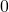for the respective probability and because we multiply all of the probabilities the overall probability would give… not very helpful! Therefore we addto all frequencies. This technique is called Laplace smoothing (or additive smoothing).
• Because the probabilities for every given word is relatively small multiplying many of those small probabilities will give us even smaller probabilities and, in the end, is likely to give us an underflow error. Therefore we are going to use the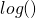function on all probabilities and instead of multiplying all of them we sum them up (btw, we still have to addto the frequencies because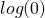is undefined!).

That was a lot of theory, let us now see the method work its magic in practice!

The original source of the data set is from here: Movie Review Data. It consists of 1,000 positive and 1,000 negative reviews. I created two files with all of the positive and negatives reviews respectively and zipped them so that they can be used directly for our purpose: Reviews.zip.

First unpack the archive and load the data into R:

pos <- readChar("data/pos.txt", file.info("data/pos.txt")$size) neg <- readChar("data/neg.txt", file.info("data/neg.txt")$size)


Bag-of-words model: the following is a very simple function to tokenize the text, i.e. separate all the words, filter out relevant stuff and in this case only retain words that consist of more than 3 characters (so stop words such as “the”, “a”, “an”, “in” are not part of the analysis):

tokenize <- function(text) {
text <- tolower(text)
text <- gsub(". ", " ", text, fixed = TRUE)
text <- gsub(": ", " ", text, fixed = TRUE)
text <- gsub("? ", " ", text, fixed = TRUE)
text <- gsub("! ", " ", text, fixed = TRUE)
text <- gsub("; ", " ", text, fixed = TRUE)
text <- gsub(", ", " ", text, fixed = TRUE)
text <- gsub("\", " ", text, fixed = TRUE)
text <- gsub("\n", " ", text, fixed = TRUE)
text <- unlist(strsplit(text, " "))
text[nchar(text) > 3]
}
pos_tokens <- tokenize(pos); neg_tokens <- tokenize(neg)


The following function calculates the log-probability of occurrence for a given token:

calc_Probs <- function(tokens) {
counts <- table(tokens) + 1
log(counts/sum(counts))
}
pos_probs <- calc_Probs(pos_tokens); neg_probs <- calc_Probs(neg_tokens)


As indicated in the text we also need a function to calculate the probability of occurrence for tokens that don’t happen to appear in the text:

calc_Prob_Rare <- function(tokens) {
counts <- table(tokens) + 1
log(1/sum(counts))
}
pos_probs_rare <- calc_Prob_Rare(pos_tokens); neg_probs_rare <- calc_Prob_Rare(neg_tokens)


Now, we are good to go! The next function calculates the positive and negative sentiment according to the process described above. Note that in the case that a word is not found in the given text the probability will be calculated taking pos_probs_rare or neg_probs_rare calculated before. Also, note that all probabilities are summed up because of the logarithm. At the end we just compare the two frequencies and return which is bigger:

calc_Sentiment <- function(review) {
test <- tokenize(review)
pos_pred <- sum(is.na(pos_probs[test])) * pos_probs_rare + sum(pos_probs[test], na.rm = TRUE)
neg_pred <- sum(is.na(neg_probs[test])) * neg_probs_rare + sum(neg_probs[test], na.rm = TRUE)
ifelse(pos_pred > neg_pred, "positive", "negative")
}
# positive
calc_Sentiment("This is a wonderful movie. I really loved it!")
##  "positive"

calc_Sentiment("I found this film so awesome, it made me cry")
##  "positive"

calc_Sentiment("I have never seen such a great movie. Best ever")
##  "positive"

# negative
calc_Sentiment("This is a horrible movie. I really hated it!")
##  "negative"

calc_Sentiment("I have never seen such crap. The director should be fired")
##  "negative"

calc_Sentiment("What should I say... This is the worst movie ever")
##  "negative"


This seems to work alright! Now, for the big finale… we are going to take two original reviews from the Internet Movie Database (IMDb), first a positive one after that a negative one:

# http://www.imdb.com/title/tt0111161/?pf_rd_m=A2FGELUUNOQJNL&pf_rd_p=2164853282&pf_rd_r=0GV6ZKG9Q9YXNSJM6QNT&pf_rd_s=center-1&pf_rd_t=15506&pf_rd_i=top&ref_=chttp_tt_1
# 10/10 -> positive
##  "positive"

# http://www.imdb.com/title/tt2071491/?pf_rd_m=A2FGELUUNOQJNL&pf_rd_p=2164859782&pf_rd_r=1ZS06QED60VF2ZC2P2EV&pf_rd_s=center-1&pf_rd_t=15506&pf_rd_i=bottom&ref_=chtbtm_tt_2
# 1/10 -> negative
##  "negative"
`

Wow, the simple piece of algorithmic wonder classified both correctly!

As you can imagine the same technique can be used for spam filtering, or categorizing texts into different areas like politics, business, sports, etc.

Sometimes it just pays to be naive…